# ConcurrentHashMap1.7和1.8的底层不同实现

https://my.oschina.net/zupengliu/blog/1930025

### 1.Hashmap和HashTable在线程安全方面的优劣？

Hashmap多线程会导致HashMap的Entry链表形成环形数据结构，一旦形成环形数据结构，Entry的next节点永远不为空，就会产生死循环获取Entry。

HashTable使用synchronized来保证线程安全，但在线程竞争激烈的情况下HashTable的效率非常低下。因为当一个线程访问HashTable的同步方法，其他线程也访问HashTable的同步方法时，会进入阻塞或轮询状态。如线程1使用put进行元素添加，线程2不但不能使用put方法添加元素，也不能使用get方法来获取元素，所以竞争越激烈效率越低。

### Hash

ConcurrentHashMap在发生hash冲突时采用了链地址法。

md4,md5,sha-hash算法也属于hash算法，又称摘要算法。

### 位运算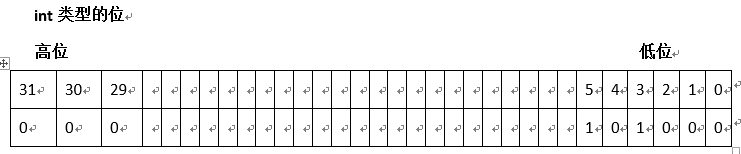2的0次方 = 1，2的1次方=2…….，以上表格代表数字 （2的5次方+2的3次方）= 40

Java实际保存int型时 正数  第31位 =0        负数：第31位=1

1. 位与  &  (1&1=1         1&0=0      0&0=0)
2. 位或  |   (1|1=1           1|0=1   0|0=0)
3. 位非  ~  （ ~1=0         ~0=1）
4. 位异或  ^   (1^1=0      1^0=1     0^0=0)
5. <<有符号左移     >>有符号的右移    >>>无符号右移  例如：8 << 2 = 32       8>>2 = 2
6. 取模的操作 a % (Math.pow(2,n)) 等价于 a&( Math.pow(2,n)-1)

### 3.  1.7中原理和实现

• ConcurrentHashMap中的数据结构

ConcurrentHashMap是由Segment数组结构和HashEntry数组结构组成。Segment实际继承自可重入锁（ReentrantLock），在ConcurrentHashMap里扮演锁的角色；HashEntry则用于存储键值对数据。一个ConcurrentHashMap里包含一个Segment数组，每个Segment里包含一个HashEntry数组，我们称之为table，每个HashEntry是一个链表结构的元素。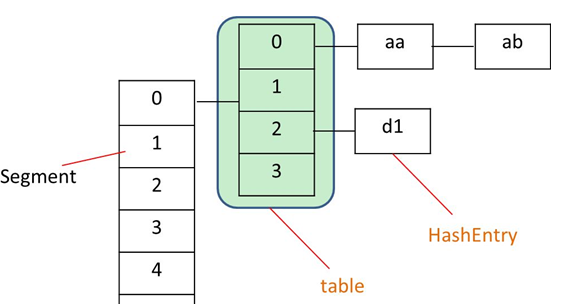1. ConcurrentHashMap实现原理是怎么样的或者问ConcurrentHashMap如何在保证高并发下线程安全的同时实现了性能提升？

• 初始化做了什么事？

initialCapacity：初始容量大小 ，默认16。

concurrencyLevel 并发度，默认16。并发度可以理解为程序运行时能够同时更新ConccurentHashMap且不产生锁竞争的最大线程数，实际上就是ConcurrentHashMap中的分段锁个数，即Segment[]的数组长度。如果并发度设置的过小，会带来严重的锁竞争问题；如果并发度设置的过大，原本位于同一个Segment内的访问会扩散到不同的Segment中，CPU cache命中率会下降，从而引起程序性能下降。

1、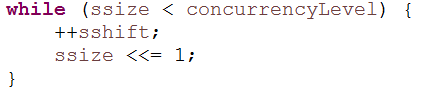2、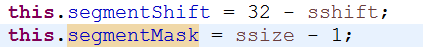3、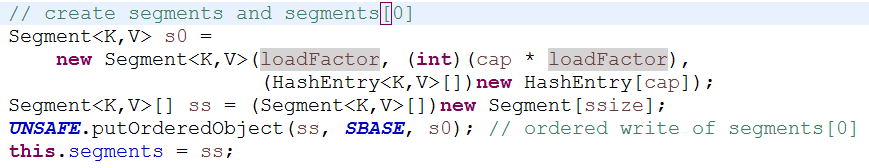4、• 在get和put操作中，是如何快速定位元素放在哪个位置的？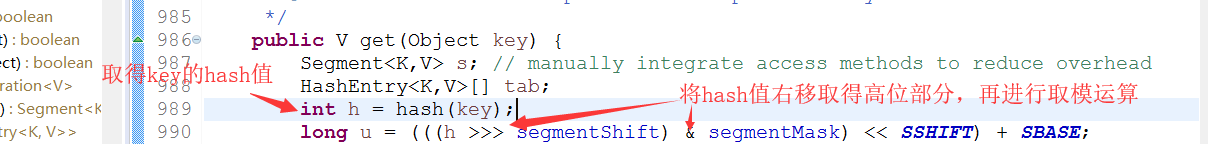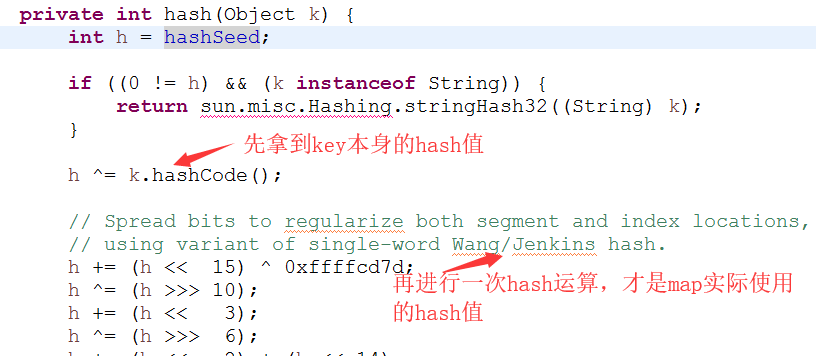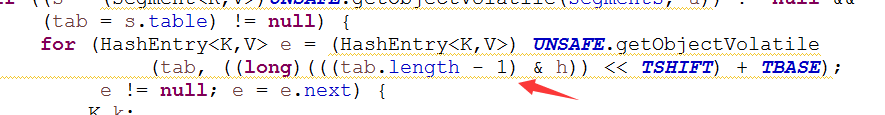• get（）方法• put()方法

1、首先定位segment，当这个segment在map初始化后，还为null，由ensureSegment方法负责填充这个segment。

1. 对Segment 加锁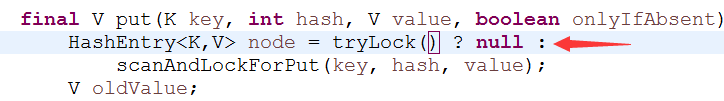3、定位所在的table元素，并扫描table下的链表，找到时：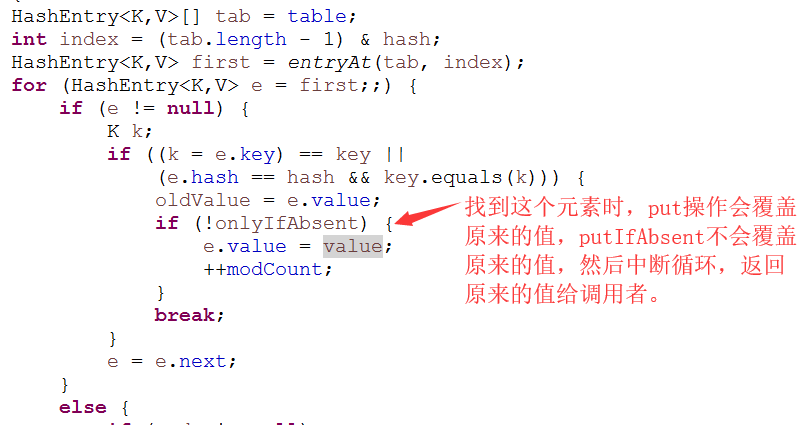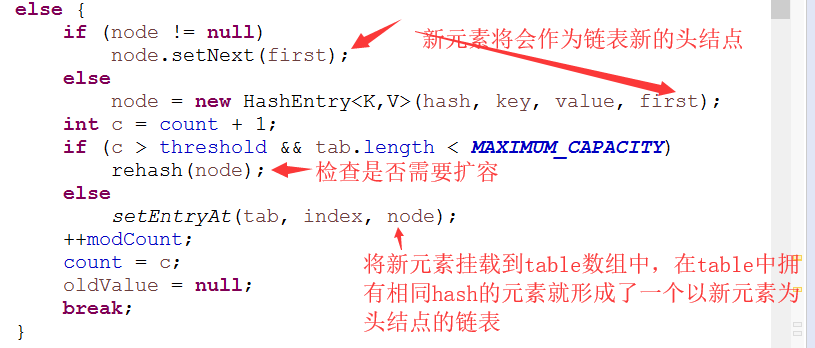• 扩容操作

Segment 不扩容，扩容下面的table数组，每次都是将数组翻倍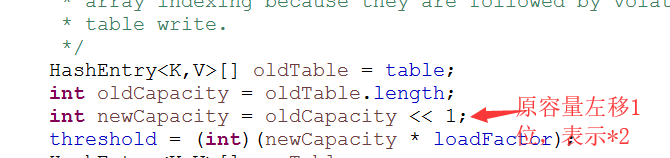Hash值 15 23 34 56 77 在table中下标 3  = 15%4 3 = 23 % 4 2 = 34%4 0 = 56%4 1 = 77 % 4

 Hash值 56 34 77 15,23 下标 0 1 2 3 4 5 6 7

• size方法

size的时候进行两次不加锁的统计，两次一致直接返回结果，不一致，重新加锁再次统计

• 弱一致性

get方法和containsKey方法都是通过对链表遍历判断是否存在key相同的节点以及获得该节点的value。但由于遍历过程中其他线程可能对链表结构做了调整，因此get和containsKey返回的可能是过时的数据，这一点是ConcurrentHashMap在弱一致性上的体现。

### 4.  1.8中的原理和实现

• 数据结构

JDK1.8的实现已经摒弃了Segment的概念，而是直接用Node数组+链表+红黑树的数据结构来实现，并发控制使用Synchronized和CAS来操作，整个看起来就像是优化过且线程安全的HashMap，虽然在JDK1.8中还能看到Segment的数据结构，但是已经简化了属性，只是为了兼容旧版本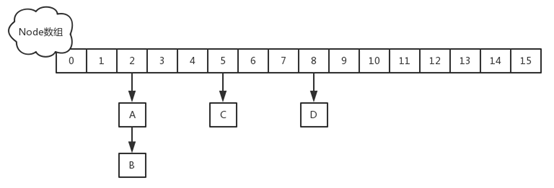// node数组最大容量：2^30=1073741824
private static final int MAXIMUM_CAPACITY = 1 << 30;
// 默认初始值，必须是2的幕数
private static final int DEFAULT_CAPACITY = 16;
//数组可能最大值，需要与toArray（）相关方法关联
static final int MAX_ARRAY_SIZE = Integer.MAX_VALUE - 8;
//并发级别，遗留下来的，为兼容以前的版本
private static final int DEFAULT_CONCURRENCY_LEVEL = 16;
// 负载因子
private static final float LOAD_FACTOR = 0.75f;
// 链表转红黑树阀值,> 8 链表转换为红黑树
static final int TREEIFY_THRESHOLD = 8;
//树转链表阀值，小于等于6（tranfer时，lc、hc=0两个计数器分别++记录原bin、新binTreeNode数量，<=UNTREEIFY_THRESHOLD 则untreeify(lo)）
static final int UNTREEIFY_THRESHOLD = 6;
static final int MIN_TREEIFY_CAPACITY = 64;
private static final int MIN_TRANSFER_STRIDE = 16;
private static int RESIZE_STAMP_BITS = 16;
// 2^15-1，help resize的最大线程数
private static final int MAX_RESIZERS = (1 << (32 - RESIZE_STAMP_BITS)) - 1;
// 32-16=16，sizeCtl中记录size大小的偏移量
private static final int RESIZE_STAMP_SHIFT = 32 - RESIZE_STAMP_BITS;
// forwarding nodes的hash值
static final int MOVED     = -1;
// 树根节点的hash值
static final int TREEBIN   = -2;
// ReservationNode的hash值
static final int RESERVED  = -3;
// 可用处理器数量
static final int NCPU = Runtime.getRuntime().availableProcessors();
//存放node的数组
transient volatile Node<K,V>[] table;
/*控制标识符，用来控制table的初始化和扩容的操作，不同的值有不同的含义
*当为负数时：-1代表正在初始化，-N代表有N-1个线程正在 进行扩容
*当为0时：代表当时的table还没有被初始化
*当为正数时：表示初始化或者下一次进行扩容的大小*/
private transient volatile int sizeCtl;


• put操作

public V put(K key, V value) {
return putVal(key, value, false);
}
/** Implementation for put and putIfAbsent */
final V putVal(K key, V value, boolean onlyIfAbsent) {
if (key == null || value == null) throw new NullPointerException();
int hash = spread(key.hashCode()); //两次hash，减少hash冲突，可以均匀分布
int binCount = 0;
for (Node<K,V>[] tab = table;;) { //对这个table进行迭代
Node<K,V> f; int n, i, fh;
//这里就是上面构造方法没有进行初始化，在这里进行判断，为null就调用initTable进行初始化，属于懒汉模式初始化
if (tab == null || (n = tab.length) == 0)
tab = initTable();
else if ((f = tabAt(tab, i = (n - 1) & hash)) == null) {//如果i位置没有数据，就直接无锁插入
if (casTabAt(tab, i, null,
new Node<K,V>(hash, key, value, null)))
break;                   // no lock when adding to empty bin
}
else if ((fh = f.hash) == MOVED)//如果在进行扩容，则先进行扩容操作
tab = helpTransfer(tab, f);
else {
V oldVal = null;
//如果以上条件都不满足，那就要进行加锁操作，也就是存在hash冲突，锁住链表或者红黑树的头结点
synchronized (f) {
if (tabAt(tab, i) == f) {
if (fh >= 0) { //表示该节点是链表结构
binCount = 1;
for (Node<K,V> e = f;; ++binCount) {
K ek;
//这里涉及到相同的key进行put就会覆盖原先的value
if (e.hash == hash &&
((ek = e.key) == key ||
(ek != null && key.equals(ek)))) {
oldVal = e.val;
if (!onlyIfAbsent)
e.val = value;
break;
}
Node<K,V> pred = e;
if ((e = e.next) == null) {  //插入链表尾部
pred.next = new Node<K,V>(hash, key,
value, null);
break;
}
}
}
else if (f instanceof TreeBin) {//红黑树结构
Node<K,V> p;
binCount = 2;
//红黑树结构旋转插入
if ((p = ((TreeBin<K,V>)f).putTreeVal(hash, key,
value)) != null) {
oldVal = p.val;
if (!onlyIfAbsent)
p.val = value;
}
}
}
}
if (binCount != 0) { //如果链表的长度大于8时就会进行红黑树的转换
if (binCount >= TREEIFY_THRESHOLD)
treeifyBin(tab, i);
if (oldVal != null)
return oldVal;
break;
}
}
}
return null;
}


1. 如果没有初始化就先调用initTable（）方法来进行初始化过程
2. 如果没有hash冲突就直接CAS插入
3. 如果还在进行扩容操作就先进行扩容
4. 如果存在hash冲突，就加锁来保证线程安全，这里有两种情况，一种是链表形式就直接遍历到尾端插入，一种是红黑树就按照红黑树结构插入，
5. 最后一个如果该链表的数量大于阈值8，就要先转换成黑红树的结构，break再一次进入循环

/**
* Initializes table, using the size recorded in sizeCtl.
*/
private final Node<K,V>[] initTable() {
Node<K,V>[] tab; int sc;
while ((tab = table) == null || tab.length == 0) {//空的table才能进入初始化操作
if ((sc = sizeCtl) < 0) //sizeCtl<0表示其他线程已经在初始化了或者扩容了，挂起当前线程
Thread.yield(); // lost initialization race; just spin
else if (U.compareAndSwapInt(this, SIZECTL, sc, -1)) {//CAS操作SIZECTL为-1，表示初始化状态
try {
if ((tab = table) == null || tab.length == 0) {
int n = (sc > 0) ? sc : DEFAULT_CAPACITY;
@SuppressWarnings("unchecked")
Node<K,V>[] nt = (Node<K,V>[])new Node<?,?>[n];//初始化
table = tab = nt;
sc = n - (n >>> 2);//记录下次扩容的大小
}
} finally {
sizeCtl = sc;
}
break;
}
}
return tab;
}


else if ((f = tabAt(tab, i = (n - 1) & hash)) == null) {
if (casTabAt(tab, i, null,
new Node<K,V>(hash, key, value, null)))
break;                   // no lock when adding to empty bin
}
/********************************/
static final <K,V> boolean casTabAt(Node<K,V>[] tab, int i,
Node<K,V> c, Node<K,V> v) {
return U.compareAndSwapObject(tab, ((long)i << ASHIFT) + ABASE, c, v);
}

/**
* Helps transfer if a resize is in progress.
* 帮助从旧的table的元素复制到新的table中
*/
final Node<K,V>[] helpTransfer(Node<K,V>[] tab, Node<K,V> f) {
Node<K,V>[] nextTab; int sc;
if (tab != null && (f instanceof ForwardingNode) &&
(nextTab = ((ForwardingNode<K,V>)f).nextTable) != null) { //新的table nextTba已经存在前提下才能帮助扩容
int rs = resizeStamp(tab.length);
while (nextTab == nextTable && table == tab &&
(sc = sizeCtl) < 0) {
if ((sc >>> RESIZE_STAMP_SHIFT) != rs || sc == rs + 1 ||
sc == rs + MAX_RESIZERS || transferIndex <= 0)
break;
if (U.compareAndSwapInt(this, SIZECTL, sc, sc + 1)) {
transfer(tab, nextTab);//调用扩容方法
break;
}
}
return nextTab;
}
return table;
}


private final void transfer(Node<K,V>[] tab, Node<K,V>[] nextTab) {
int n = tab.length, stride;
// 每核处理的量小于16，则强制赋值16
if ((stride = (NCPU > 1) ? (n >>> 3) / NCPU : n) < MIN_TRANSFER_STRIDE)
stride = MIN_TRANSFER_STRIDE; // subdivide range
if (nextTab == null) {            // initiating
try {
@SuppressWarnings("unchecked")
Node<K,V>[] nt = (Node<K,V>[])new Node<?,?>[n << 1];        //构建一个nextTable对象，其容量为原来容量的两倍
nextTab = nt;
} catch (Throwable ex) {      // try to cope with OOME
sizeCtl = Integer.MAX_VALUE;
return;
}
nextTable = nextTab;
transferIndex = n;
}
int nextn = nextTab.length;
// 连接点指针，用于标志位（fwd的hash值为-1，fwd.nextTable=nextTab）
ForwardingNode<K,V> fwd = new ForwardingNode<K,V>(nextTab);
// 当advance == true时，表明该节点已经处理过了
boolean advance = true;
boolean finishing = false; // to ensure sweep before committing nextTab
for (int i = 0, bound = 0;;) {
Node<K,V> f; int fh;
// 控制 --i ,遍历原hash表中的节点
int nextIndex, nextBound;
if (--i >= bound || finishing)
else if ((nextIndex = transferIndex) <= 0) {
i = -1;
}
// 用CAS计算得到的transferIndex
else if (U.compareAndSwapInt
(this, TRANSFERINDEX, nextIndex,
nextBound = (nextIndex > stride ?
nextIndex - stride : 0))) {
bound = nextBound;
i = nextIndex - 1;
}
}
if (i < 0 || i >= n || i + n >= nextn) {
int sc;
// 已经完成所有节点复制了
if (finishing) {
nextTable = null;
table = nextTab;        // table 指向nextTable
sizeCtl = (n << 1) - (n >>> 1);     // sizeCtl阈值为原来的1.5倍
return;     // 跳出死循环，
}
// CAS 更扩容阈值，在这里面sizectl值减一，说明新加入一个线程参与到扩容操作
if (U.compareAndSwapInt(this, SIZECTL, sc = sizeCtl, sc - 1)) {
if ((sc - 2) != resizeStamp(n) << RESIZE_STAMP_SHIFT)
return;
finishing = advance = true;
i = n; // recheck before commit
}
}
// 遍历的节点为null，则放入到ForwardingNode 指针节点
else if ((f = tabAt(tab, i)) == null)
advance = casTabAt(tab, i, null, fwd);
// f.hash == -1 表示遍历到了ForwardingNode节点，意味着该节点已经处理过了
// 这里是控制并发扩容的核心
else if ((fh = f.hash) == MOVED)
else {
// 节点加锁
synchronized (f) {
// 节点复制工作
if (tabAt(tab, i) == f) {
Node<K,V> ln, hn;
// fh >= 0 ,表示为链表节点
if (fh >= 0) {
// 构造两个链表  一个是原链表  另一个是原链表的反序排列
int runBit = fh & n;
Node<K,V> lastRun = f;
for (Node<K,V> p = f.next; p != null; p = p.next) {
int b = p.hash & n;
if (b != runBit) {
runBit = b;
lastRun = p;
}
}
if (runBit == 0) {
ln = lastRun;
hn = null;
}
else {
hn = lastRun;
ln = null;
}
for (Node<K,V> p = f; p != lastRun; p = p.next) {
int ph = p.hash; K pk = p.key; V pv = p.val;
if ((ph & n) == 0)
ln = new Node<K,V>(ph, pk, pv, ln);
else
hn = new Node<K,V>(ph, pk, pv, hn);
}
// 在nextTable i 位置处插上链表
setTabAt(nextTab, i, ln);
// 在nextTable i + n 位置处插上链表
setTabAt(nextTab, i + n, hn);
// 在table i 位置处插上ForwardingNode 表示该节点已经处理过了
setTabAt(tab, i, fwd);
// advance = true 可以执行--i动作，遍历节点
}
// 如果是TreeBin，则按照红黑树进行处理，处理逻辑与上面一致
else if (f instanceof TreeBin) {
TreeBin<K,V> t = (TreeBin<K,V>)f;
TreeNode<K,V> lo = null, loTail = null;
TreeNode<K,V> hi = null, hiTail = null;
int lc = 0, hc = 0;
for (Node<K,V> e = t.first; e != null; e = e.next) {
int h = e.hash;
TreeNode<K,V> p = new TreeNode<K,V>
(h, e.key, e.val, null, null);
if ((h & n) == 0) {
if ((p.prev = loTail) == null)
lo = p;
else
loTail.next = p;
loTail = p;
++lc;
}
else {
if ((p.prev = hiTail) == null)
hi = p;
else
hiTail.next = p;
hiTail = p;
++hc;
}
}
// 扩容后树节点个数若<=6，将树转链表
ln = (lc <= UNTREEIFY_THRESHOLD) ? untreeify(lo) :
(hc != 0) ? new TreeBin<K,V>(lo) : t;
hn = (hc <= UNTREEIFY_THRESHOLD) ? untreeify(hi) :
(lc != 0) ? new TreeBin<K,V>(hi) : t;
setTabAt(nextTab, i, ln);
setTabAt(nextTab, i + n, hn);
setTabAt(tab, i, fwd);
}
}
}
}
}
}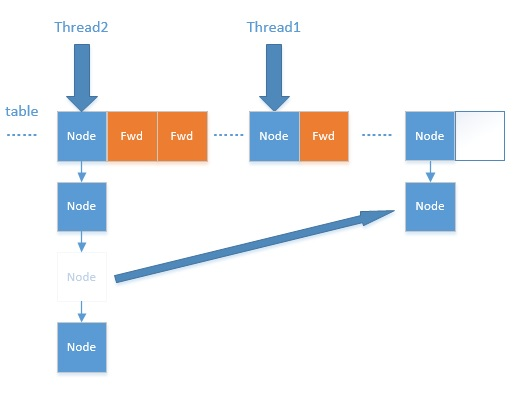private final void treeifyBin(Node<K,V>[] tab, int index) {
Node<K,V> b; int n, sc;
if (tab != null) {
//如果整个table的数量小于64，就扩容至原来的一倍，不转红黑树了
//因为这个阈值扩容可以减少hash冲突，不必要去转红黑树
if ((n = tab.length) < MIN_TREEIFY_CAPACITY)
tryPresize(n << 1);
else if ((b = tabAt(tab, index)) != null && b.hash >= 0) {
synchronized (b) {
if (tabAt(tab, index) == b) {
TreeNode<K,V> hd = null, tl = null;
for (Node<K,V> e = b; e != null; e = e.next) {
//封装成TreeNode
TreeNode<K,V> p =
new TreeNode<K,V>(e.hash, e.key, e.val,
null, null);
if ((p.prev = tl) == null)
hd = p;
else
tl.next = p;
tl = p;
}
//通过TreeBin对象对TreeNode转换成红黑树
setTabAt(tab, index, new TreeBin<K,V>(hd));
}
}
}
}
}


private final void addCount(long x, int check) {
CounterCell[] as; long b, s;
//更新baseCount，table的数量，counterCells表示元素个数的变化
if ((as = counterCells) != null ||
!U.compareAndSwapLong(this, BASECOUNT, b = baseCount, s = b + x)) {
CounterCell a; long v; int m;
boolean uncontended = true;
if (as == null || (m = as.length - 1) < 0 ||
(a = as[ThreadLocalRandom.getProbe() & m]) == null ||
!(uncontended =
U.compareAndSwapLong(a, CELLVALUE, v = a.value, v + x))) {
return;
}
if (check <= 1)
return;
s = sumCount();
}
//check>=0表示需要进行扩容操作
if (check >= 0) {
Node<K,V>[] tab, nt; int n, sc;
while (s >= (long)(sc = sizeCtl) && (tab = table) != null &&
(n = tab.length) < MAXIMUM_CAPACITY) {
int rs = resizeStamp(n);
if (sc < 0) {
if ((sc >>> RESIZE_STAMP_SHIFT) != rs || sc == rs + 1 ||
sc == rs + MAX_RESIZERS || (nt = nextTable) == null ||
transferIndex <= 0)
break;
if (U.compareAndSwapInt(this, SIZECTL, sc, sc + 1))
transfer(tab, nt);
}
//当前线程发起库哦哦让操作，nextTable=null
else if (U.compareAndSwapInt(this, SIZECTL, sc,
(rs << RESIZE_STAMP_SHIFT) + 2))
transfer(tab, null);
s = sumCount();
}
}
}


put的流程现在已经分析完了，你可以从中发现，他在并发处理中使用的是乐观锁，当有冲突的时候才进行并发处理，而且流程步骤很清晰，但是细节设计的很复杂，毕竟多线程的场景也复杂

• get操作

public V get(Object key) {
Node<K,V>[] tab; Node<K,V> e, p; int n, eh; K ek;
int h = spread(key.hashCode()); //计算hash,再散列
if ((tab = table) != null && (n = tab.length) > 0 &&
(e = tabAt(tab, (n - 1) & h)) != null) {//读取首节点的Node元素
if ((eh = e.hash) == h) { //如果该节点就是首节点就返回
if ((ek = e.key) == key || (ek != null && key.equals(ek)))
return e.val;
}
//hash值为负值表示正在扩容，这个时候查的是ForwardingNode的find方法来定位到nextTable来
//查找，查找到就返回
else if (eh < 0)
return (p = e.find(h, key)) != null ? p.val : null;
while ((e = e.next) != null) {//既不是首节点也不是ForwardingNode，那就往下遍历
if (e.hash == h &&
((ek = e.key) == key || (ek != null && key.equals(ek))))
return e.val;
}
}
return null;
}


ConcurrentHashMap的get操作的流程很简单，也很清晰，可以分为三个步骤来描述

1. 计算hash值，定位到该table索引位置，如果是首节点符合就返回
2. 如果遇到扩容的时候，会调用标志正在扩容节点ForwardingNode的find方法，查找该节点，匹配就返回
3. 以上都不符合的话，就往下遍历节点，匹配就返回，否则最后就返回null
• size操作

public int size() {
long n = sumCount();
return ((n < 0L) ? 0 :
(n > (long)Integer.MAX_VALUE) ? Integer.MAX_VALUE :
(int)n);
}
final long sumCount() {
CounterCell[] as = counterCells; CounterCell a; //变化的数量
long sum = baseCount;
if (as != null) {
for (int i = 0; i < as.length; ++i) {
if ((a = as[i]) != null)
sum += a.value;
}
}
return sum;
}


### 5.总结

1. JDK1.8取消了segment数组，直接用table保存数据，锁的粒度更小，减少并发冲突的概率。
2. JDK1.8存储数据时采用了链表+红黑树的形式，纯链表的形式时间复杂度为O(n)，红黑树则为O（logn），性能提升很大。什么时候链表转红黑树？当key值相等的元素形成的链表中元素个数超过8个的时候。
3. JDK1.8的实现降低锁的粒度，JDK1.7版本锁的粒度是基于Segment的，包含多个HashEntry，而JDK1.8锁的粒度就是HashEntry（首节点）
4. JDK1.8版本的数据结构变得更加简单，使得操作也更加清晰流畅，因为已经使用synchronized来进行同步，所以不需要分段锁的概念，也就不需要Segment这种数据结构了，由于粒度的降低，实现的复杂度也增加了
5. JDK1.8使用红黑树来优化链表，基于长度很长的链表的遍历是一个很漫长的过程，而红黑树的遍历效率是很快的，代替一定阈值的链表，这样形成一个最佳拍档
6. JDK1.8为什么使用内置锁synchronized来代替重入锁ReentrantLock，我觉得有以下几点
1. 因为粒度降低了，在相对而言的低粒度加锁方式，synchronized并不比ReentrantLock差，在粗粒度加锁中ReentrantLock可能通过Condition来控制各个低粒度的边界，更加的灵活，而在低粒度中，Condition的优势就没有了
2. JVM的开发团队从来都没有放弃synchronized，而且基于JVM的synchronized优化空间更大，使用内嵌的关键字比使用API更加自然
3. 在大量的数据操作下，对于JVM的内存压力，基于API的ReentrantLock会开销更多的内存，虽然不是瓶颈，但是也是一个选择依据
03-222184
10-121361
08-13391
03-027869
07-01896
09-281129
03-222484
09-031万+
08-08273
04-10718
04-243020
02-201384
08-15185
04-096580
09-03912
01-25178
06-13946
04-111772
08-16205

### “相关推荐”对你有帮助么？

•非常没帮助
•没帮助
•一般
•有帮助
•非常有帮助

©️2022 CSDN 皮肤主题：编程工作室 设计师：CSDN官方博客被折叠的  条评论 为什么被折叠?到【灌水乐园】发言点击重新获取扫码支付1.余额是钱包充值的虚拟货币，按照1:1的比例进行支付金额的抵扣。
2.余额无法直接购买下载，可以购买VIP、C币套餐、付费专栏及课程。余额充值